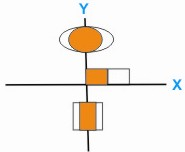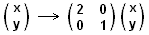Question: What is Stretch ? Answer: A stretch is a transformation of the plane in which all points move at right angles to a fixed line, a distance proportional to their distance from the line to start with.The example shows the effect of a stretch from the y-axis on a variety of shapes where the scale factor of the stretch is 2. all the x-co ordinates are doubled but the y-coordinates are unchanged. This transformation is represented in matrix form as